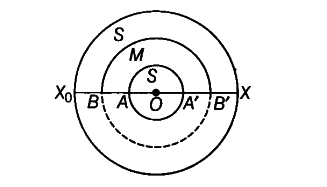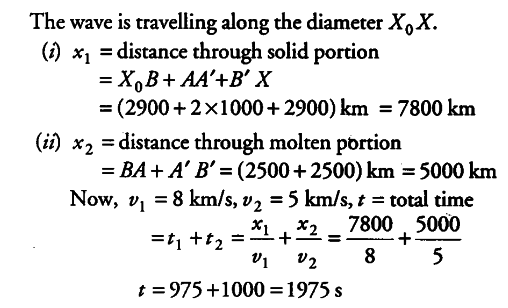# The earth has a radius of 6400 km

The earth has a radius of 6400 km. The inner core of 1000 km radius is solid. Outside it, there is a region from 1000 km to a radius of 3500 km which is in molten state. Then, again from 3500 km to 6400 km the earth is solid. Only longitudinal § waves can travel inside a liquid. Assume that the P wave has a speed of 8 km \${{s}^{-1}}\$ in solid parts and of 5 km \${{s}^{-1}}\$ in liquid parts of the earth. An earthquake occurs at some place close to the surface of the earth. Calculate the time after which it will be recorded in a seismometer at a diametrically opposite point on the earth if wave travels along diameter?

Consider the diagram shown below